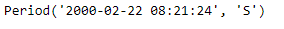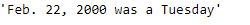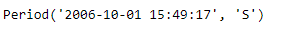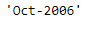Skip to content
Related Articles
Python | Pandas Period.strftime
• Last Updated : 06 Jan, 2019

Python is a great language for doing data analysis, primarily because of the fantastic ecosystem of data-centric python packages. Pandas is one of those packages and makes importing and analyzing data much easier.

Pandas` Period.strftime()` function returns the string representation of the Period, depending on the selected format. format must be a string containing one or several directives. The method recognizes the same directives as the `time.strftime()` function of the standard Python distribution, as well as the specific additional directives %f, %F, %q. (formatting & docs originally from scikits.timeries)

Syntax : Period.strftime()

Parameters : format

Return : string

Example #1: Use `Period.strftime()` function to return the value of the given period in a specified format.

 `# importing pandas as pd``import` `pandas as pd`` ` `# Create the Period object``prd ``=` `pd.Period(freq ``=``'S'``, year ``=` `2000``, month ``=` `2``, day ``=` `22``, ``                        ``hour ``=` `8``, minute ``=` `21``, second ``=` `24``)`` ` `# Print the Period object``print``(prd)`

Output :Now we will use the `Period.strftime()` function to return the given period in (‘%b. %d, %Y was a %A’) format.

Note : `'%b'` is month name, `%d` is day of the month, `%Y` is year and `%A` is weekday name.

 `# return the period in specified format.``prd.strftime(``'% b. % d, % Y was a % A'``)`

Output :As we can see in the output, the `Period.strftime()` function has returned the value of the given period in the specified format.

Example #2: Use `Period.strftime()` function to return the value of the given period in a specified format.

 `# importing pandas as pd``import` `pandas as pd`` ` `# Create the Period object``prd ``=` `pd.Period(freq ``=``'S'``, year ``=` `2006``, month ``=` `10``, ``               ``hour ``=` `15``, minute ``=` `49``, second ``=` `17``)`` ` `# Print the object``print``(prd)`

Output :Now we will use the `Period.strftime()` function to return the given period in (‘%b-%Y’) format.

Note : `'%b'` is month name, `%Y` is year

 `# return the period in specified format.``prd.strftime(``'% b-% Y'``)`

Output :As we can see in the output, the `Period.strftime()` function has returned the value of the given period in the specified format.

Attention geek! Strengthen your foundations with the Python Programming Foundation Course and learn the basics.

To begin with, your interview preparations Enhance your Data Structures concepts with the Python DS Course. And to begin with your Machine Learning Journey, join the Machine Learning – Basic Level Course

My Personal Notes arrow_drop_up